Question

# Below part D is a multiple-flash picture of a ball rolling on a smooth surface, left...

Below part D is a multiple-flash picture of a ball rolling on a smooth surface, left to right. The flashes come at equal time intervals.

(A) Is the ball moving at constant velocity? How do you know?

(B) If not, where is it fastest?

(C) Where is the acceleration (if any) greatest in magnitude?

(D) Do acceleration and velocity have the same sign anywhere here? If so, where?

o     o     o     o     o o o o o o o o

here,

a)

from the pictures we can see that the ball distance traveled in each second decrease

so, it is not moving at constant velocity

b)

initially the ball is fastest

c)

the acceleration is greater in magnitude when the distance traveled by ball in each time interval decreases

d)

No, as the distance traveled is decreased

the ball will slow down

the acceleration is in opposite direction to the velocity

#### Earn Coins

Coins can be redeemed for fabulous gifts.

Similar Homework Help Questions
• ### A mass on a spring vibrates horizontally on a smooth level surface. Its equation of motion...

A mass on a spring vibrates horizontally on a smooth level surface. Its equation of motion is x(t) = 8sint where t is in seconds and x in centimeters a) find the velocity and acceleration at time t b) find the position, velocity and acceleration of the mass at time t = 2pi / 3 - Answer must be exact values, no decimals, i.e use the unit circle - please include units c) In what direction is it moving at...

• ### 4 and 5 4) The diagrams below show a ball swinging on a string. The diagrams...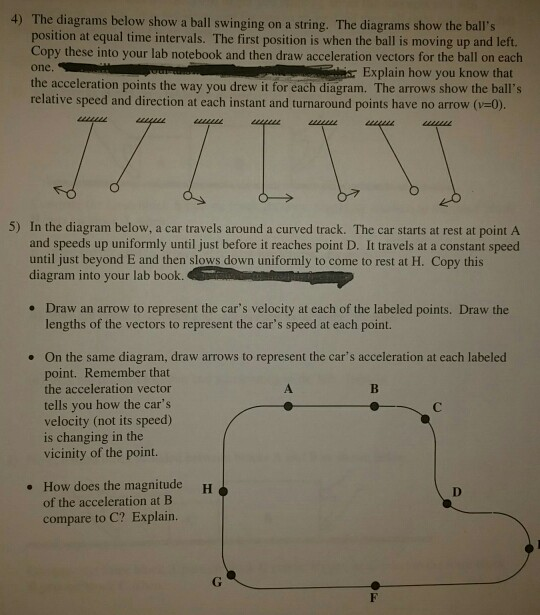4 and 5 4) The diagrams below show a ball swinging on a string. The diagrams show the ball's position at equal time intervals. The first position is when the ball is moving up and left. Copy these into your lab notebook and then draw acceleration vectors for the ball on each one the acceleration points the way you drew it for each diagram. The arrows show the ball's relative speed and direction at each instant and turnaround points have...

• ### Rolling and slipping: A relative motion approach Mech Name HW-45 6. A cylinder of radius R...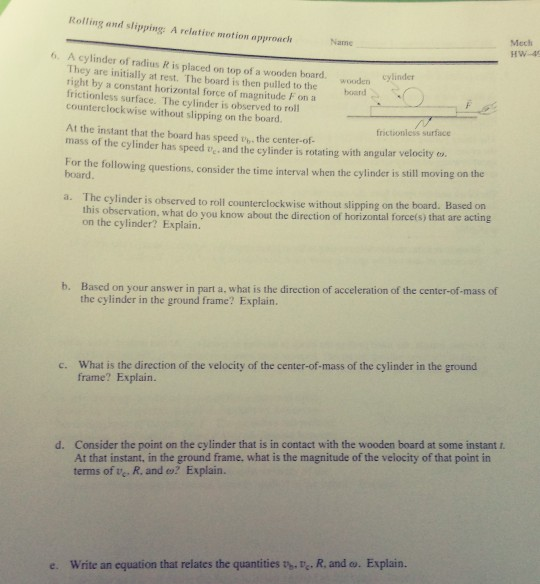Rolling and slipping: A relative motion approach Mech Name HW-45 6. A cylinder of radius R is placed on top of a wooden board. They are initially at rest. The board is then pulled to the right by a constant horizontal force of magnitude F on a frictionless surface, The cvlinder is observed to roll counterclockwise without slipping cylinder wooden board on the board frictioniess surface At the instant that the board has speed vh, the center-of- mass of the...

• ### 4 and 5. Part C question 6 is not relevant to question 4 and 5 despite...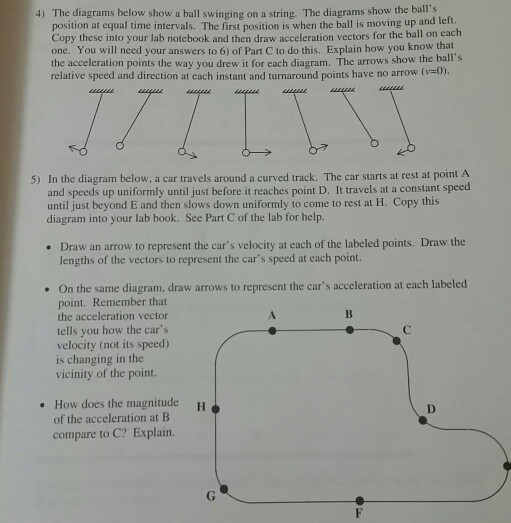4 and 5. Part C question 6 is not relevant to question 4 and 5 despite what the question says. The diagrams below show a ball swinging on a string. The diagrams show the ball's position at equal time intervals, The first position is when the ball is moving up and leit Copy these into your lab notebook and then draw acceleration vectors for the ball on each one. You will need your answers to 6) of Part C to...

• ### Part 1: A DVD is initially at rest so that the line PQ on the disc's surface is along the +x-axis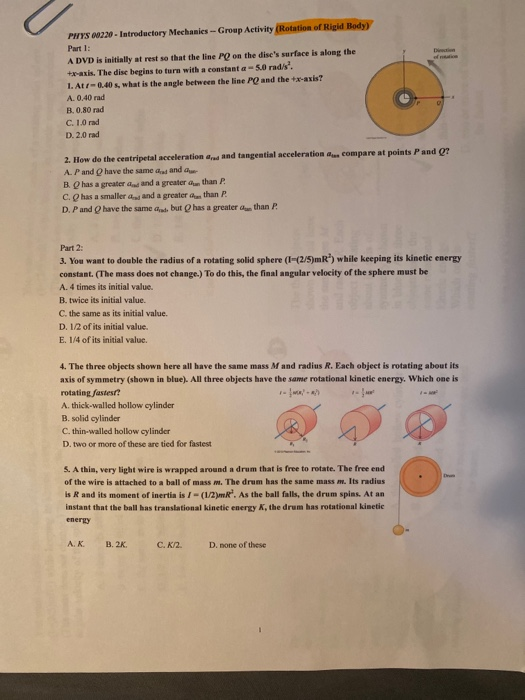Part 1: A DVD is initially at rest so that the line PQ on the disc's surface is along the +x-axis. The disc begins to turn with a constant α = 5.0 rad/s2. .1. At-0.40s, what is the angle between the line PQ and the +x-axis? A. 0.40 rad B. 0.80 rad C. 1.0 rad D. 2.0 rad  2. How do the centripetal acceleration and tangential acceleration compare at points P and Q?Part 2: 3. You want to double the radius of a rotating...

• ### Part Al Select the best answer of the following multiple choice questions (32 Points), just circle...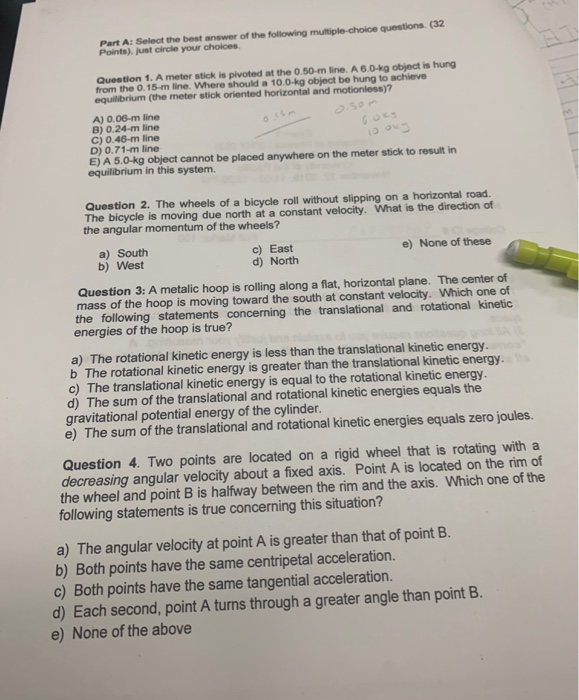Part Al Select the best answer of the following multiple choice questions (32 Points), just circle your choices Question 1. A meter stick is pivoted at the 0.50-m line. A 6.0 kg object is hung from the 0.15-m line. Where should a 10.0 kg object be hung to achieve equilibrium (the meter stick oriented horizontal and motionless)? A) 0.06-m line B) 0.24-m line C) 0.46-m line D) 0.71-m line E) A 5.0 kg object cannot be placed anywhere on the...

• ### Bob places a neutrally charged conducting ball next to a large, charged plate. The conducting ball...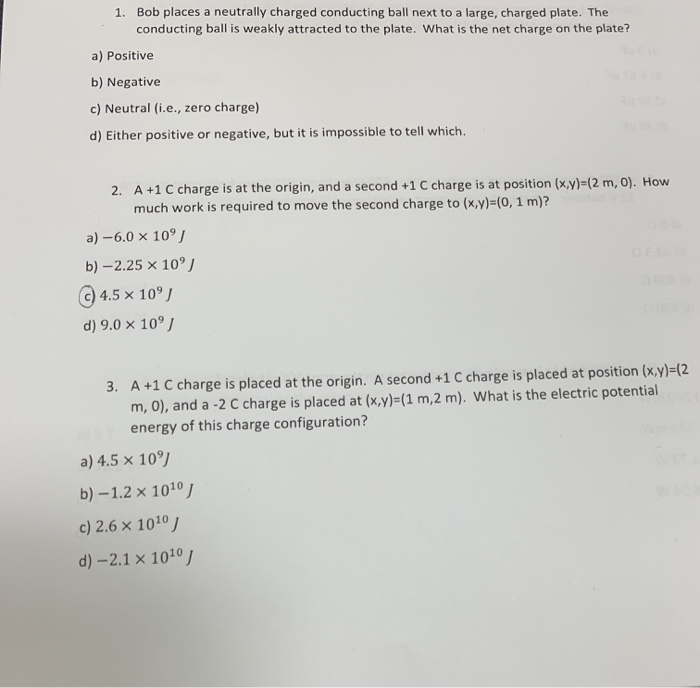Bob places a neutrally charged conducting ball next to a large, charged plate. The conducting ball is weakly attracted to the plate. What is the net charge on the plate? 1. a) Positive b) Negative c) Neutral (i.e., zero charge) d) Either positive or negative, but it is impossible to tell which A +1 C charge is at the origin, and a second +1 C charge is at position (x,y)-(2 m, 0). How much work is required to move the...

• ### Question Completion Status: QUESTION 1 In the figure below, a disk of radius R rolls on the horiz...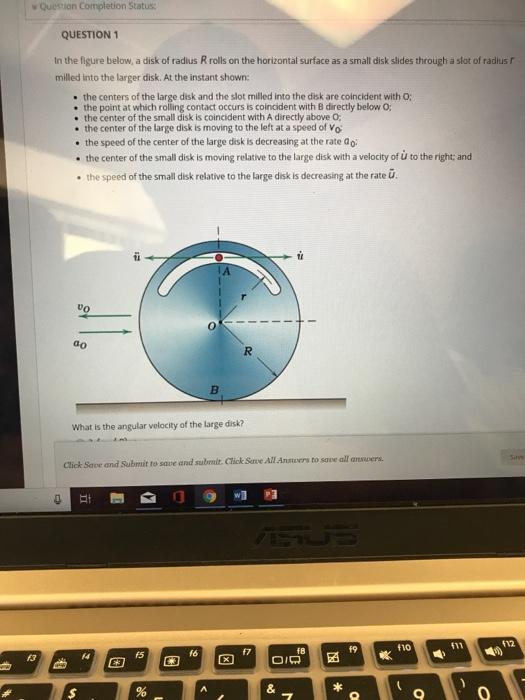i dont know how to do questions 1-6 Question Completion Status: QUESTION 1 In the figure below, a disk of radius R rolls on the horizontal surface as a small disk slides through a slot of radius r milled into the larger disk. At the instant shown: e the centers of the large disk and the slot milled into the disk are coincident with O; the point at which rolling contact occurs is coincident with B directly below O; the...

• ### PLease help me with these two multiple choice questions. only help if you know how to...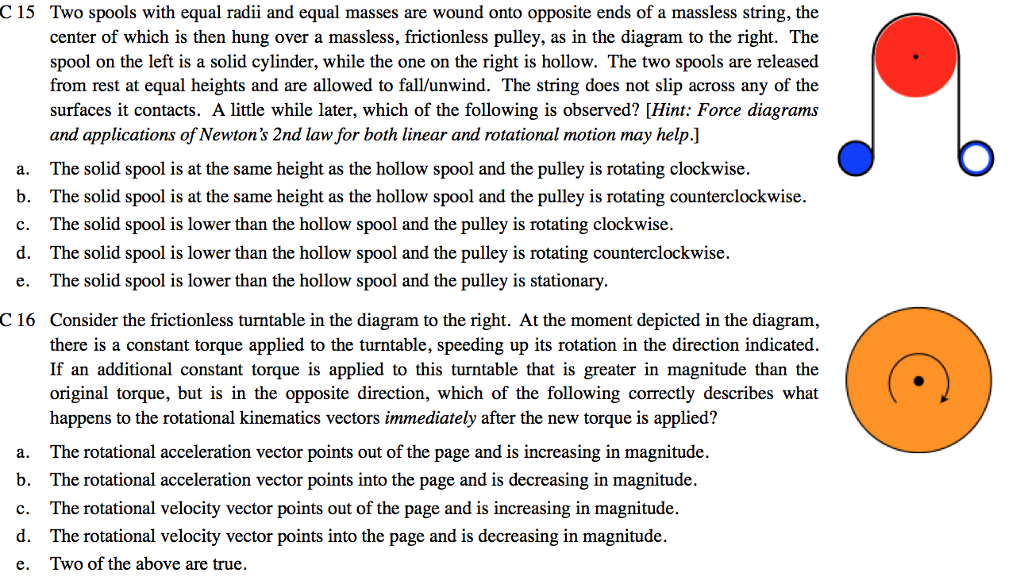PLease help me with these two multiple choice questions. only help if you know how to do it 100% and please explain the reason of choosing answer and draw if nessesary. Please write clearly C 15 Two spools with equal radii and equal masses are wound onto opposite ends of a massless string, the center of which is then hung over a massless, frictionless pulley, as in the diagram to the right. The spool on the left is a solid...

• ### need help for answering all questions and in need of an explanation for each answer. Physics...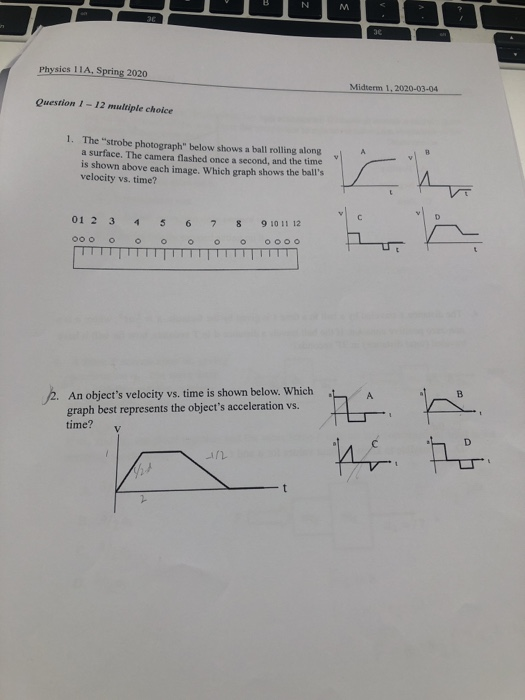need help for answering all questions and in need of an explanation for each answer. Physics 11A, Spring 2020 Midterm 1.2020-03-04 Question 1- 12 multiple choice 1. The "strobe photograph" below shows a ball rolling along a surface. The camera flashed once a second, and the time is shown above each image. Which graph shows the ball's velocity vs. time? 01 2 3 4 5 6 7 8 9 10 11 12 OOO OOO OOOO L 2. An object's velocity...